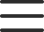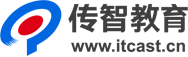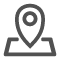# C/C++：编写高效C语言的四大绝招

C语言是很多程序猿的入门语言，而且C语言也是一门用不过时的语言。编写高效简洁的C语言代码，是许多软件工程师追求的目标。今天传智播客C/C++培训专家针对编程工作中的一些体会和经验给大家做相关的阐述。

#define LEN 32

char string1 [LEN];

memset (string1, 0, LEN);

strcpy (string1, "This is a example!!");

const char string2[LEN] ="This is a example!";

char * cp;

cp = string2 ;

int I , j;

for (I = 1 ;I<=100; I ++)

{

j += I;

}

int I;

I = (100 * (1+100)) / 2;

int I,J;

I = 257 / 8;

J = 456 % 32;

int I,J;

I = 257 >>3;

J = 456 - (456 >> 4 << 4);

a=a*4;

b=b/4;

a=a<<2;

b=b>>2;

... ...

char string1,string2;

int i;

for (i =0 ;i<1024;i++)

*(string2 + i) = *(string1 + i)

#ifdef _PC_

int I;

for (I =0 ;I<1024;I++)

*(string2 + I) = *(string1 + I);

#else

#ifdef _ARM_

__asm

{

MOV R0,string1

MOV R1,string2

MOV R2,#0

loop:

LDMIA R0!, [R3-R11]

STMIA R1!, [R3-R11]

ADD R2,R2,#8

CMP R2, #400

BNE loop

}

#endif